• Home >
• Winter 2019-2020
• Thursday 13.02.2020, 2pm, MNO 5A

Guillaume Maillard (Université Paris-Sud), Aggregated hold-out

Abstract: Aggregated hold-out (Agghoo) is a hyperparameter aggregation method which averages learning rules selected by hold-out (i.e cross-validation with 1 split). Theoretical guarantees on Agghoo ensure that one can use it safely: for a convex risk, at worse, Agghoo performs like the hold-out. For the hold-out, oracle inequalities are known for bounded losses, as in binary classification. We show that classical methods can be extended, under appropriate assumptions, to some unbounded risk-minimization problems. In particular, we obtain an oracle inequality in sparse linear regression with Huber loss, without requiring the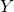variable to be bounded or using truncation. To further investigate the effects of aggregation on performance, we conduct some numerical experiments. They show that aggregation brings a significant improvement over the hold-out. Compared to cross-validation, Agghoo appears to perform better when the intrinsic dimension is sufficiently high, and when there are correlations between predictive and noise covariates.

• Thursday 06.02.2020, 2pm, MNO 5A

Richard Nickl (University of Cambridge)On Bayesian solutions of some statistical inverse boundary value problems

Abstract: We discuss Bayesian inference in a class of statistical non-linear inverse problems arising with partial differential equations (PDEs): The main mathematical idea behind non-invasive tomography methods is related to the fact that observations of boundary values of the solutions of certain PDEs can in certain cases determine the parameters governing the dynamics of the PDE also in the interior of the domain in question. The parameter to data maps in such settings are typically non-linear, as with the Calderon problem (relevant in electric impedance tomography) or with non-Abelian X-ray transforms (relevant in neutron spin tomography). Real world discrete data in such settings carries statistical noise, and Bayesian inversion methodology has been extremely popular in computational and applied mathematics in the last decade after seminal contributions by Andrew Stuart (2010) and others. In this talk we will discuss recent progress which provides rigorous statistical guarantees for such inversion algorithms in the large sample/small noise limit.

• Friday, 31.01.2020, 10:35am, MNO 5A

Xiao Fang (The Chinese University of Hong Kong)Wasserstein-2 bounds in normal approximations under local dependence with applications to strong embeddings

Abstract: We obtain a general bound for the Wasserstein-2 distance in normal approximation for sums of locally dependent random variables. The proof is based on an asymptotic expansion for expectations of second-order differentiable functions of the sum. We apply the main result to obtain Wasserstein-2 bounds in normal approximation for sums of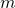-dependent random variables, U-statistics and subgraph counts in the Erdös-Rényi random graph. We also discuss an application to strong embeddings.

• Thursday 30.01.2020, 2pm, MNO 5A

Alexandre Moesching (University of Bern)Order Constraints in Nonparametric Regression

Abstract: Imposing a nonparametric qualitative constraint in a statistical model has shown its benefit on several occasions, for example in circumstances where a parametric model is hard to justify but a qualitative constraint on the distribution is natural. We consider a stochastic ordering constraints on an unknown family of distributions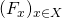, with a fixed subset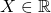, and discuss nonparametric estimation procedures based on a sample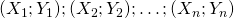such that, conditional on the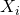the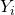are independent random variables with distribution functions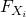.